Next: Free Electron Motion Up: Relativistic Electron Theory Previous: Dirac Equation

# Lorentz Invariance of Dirac Equation

Consider two inertial frames,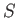and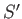. Let the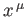and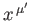be the space-time coordinates of a given event in each frame, respectively. These coordinates are related via a Lorentz transformation, which takes the general form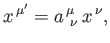(11.52)

where the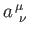are real numerical coefficients that are independent of the. We also have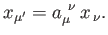(11.53)

Now, because [see Equation (11.5)]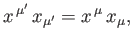(11.54)

it follows that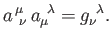(11.55)

Moreover, it is easily shown that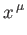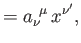(11.56)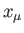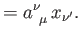(11.57)

By definition, a 4-vector,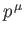, has analogous transformation properties to the. Thus,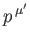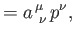(11.58)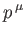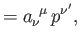(11.59)

et cetera.

In frame, the Dirac equation is written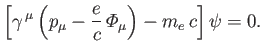(11.60)

Let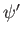be the wavefunction in frame. Suppose that(11.61)

whereis a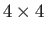transformation matrix that is independent of the. (Hence,commutes with the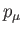and the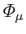.) Multiplying Equation (11.60) by, we obtain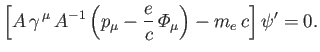(11.62)

Hence, given that theandare the covariant components of 4-vectors, we obtain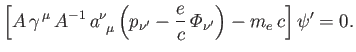(11.63)

Suppose that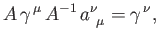(11.64)

which is equivalent to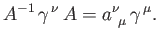(11.65)

Here, we have assumed that the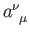commute withand the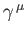(because they are just numbers). If Equation (11.64) holds then Equation (11.63) becomes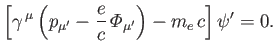(11.66)

A comparison of this equation with Equation (11.60) reveals that the Dirac equation takes the same form in framesand. In other words, the Dirac equation is Lorentz invariant. Incidentally, it is clear from Equations (11.60) and (11.66) that thematrices are the same in all inertial frames.

It remains to find a transformation matrixthat satisfies Equation (11.65). Consider an infinitesimal Lorentz transformation, for which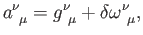(11.67)

where the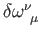are real numerical coefficients that are independent of the, and are also small compared to unity. To first order in small quantities, Equation (11.55) yields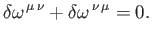(11.68)

Each of the six independent non-vanishing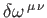generates a particular infinitesimal Lorentz transformation. For instance,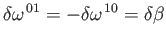(11.69)

for a transformation to a coordinate system moving with a velocity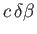along the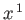-direction. Furthermore,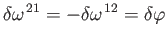(11.70)

for a rotation through an angle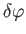about the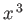-axis, and so on.

Let us write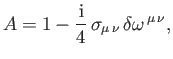(11.71)

where the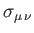are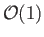matrices. To first order in small quantities,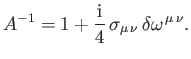(11.72)

Moreover, it follows from Equation (11.68) that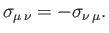(11.73)

To first order in small quantities, Equations (11.65), (11.67), (11.71), and (11.72) yield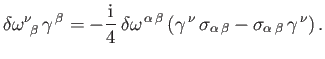(11.74)

Hence, making use of the symmetry property (11.68), we obtain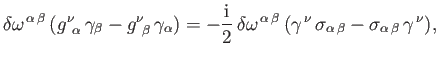(11.75)

where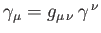. Because this equation must hold for arbitrary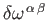, we deduce that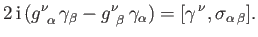(11.76)

Making use of the anti-commutation relations (11.29), it can be shown that a suitable solution of the previous equation is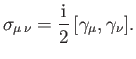(11.77)

(See Exercise 7.) Hence,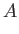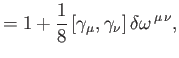(11.78)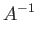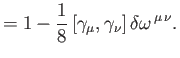(11.79)

Now that we have found the correct transformation rules for an infinitesimal Lorentz transformation, we can easily find those for a finite transformation by building it up from a large number of successive infinitesimal transforms . (See Exercises 8 and 9.)

Making use of Equation (11.34), as well as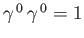, the Hermitian conjugate of Equation (11.78) can be shown to take the form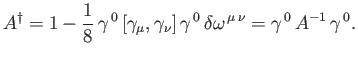(11.80)

Hence, Equation (11.65) yields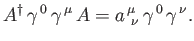(11.81)

It follows that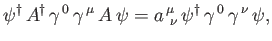(11.82)

or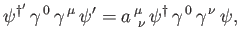(11.83)

which implies that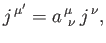(11.84)

where the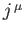are defined in Equation (11.46). This proves that thetransform as the contravariant components of a 4-vector.Next: Free Electron Motion Up: Relativistic Electron Theory Previous: Dirac Equation
Richard Fitzpatrick 2016-01-22